Function Repository Resource:

# ConvertStringsToSymbols

Convert strings inside an expression to symbols without evaluating them further

Contributed by: Sjoerd Smit
 ResourceFunction["ConvertStringsToSymbols"][expr, str] replaces all instances of str in expr with the symbol of that name. ResourceFunction["ConvertStringsToSymbols"][expr, str→"context`"] creates the symbol in a given context. ResourceFunction["ConvertStringsToSymbols"][expr, {spec1,spec2,…}] replaces multiple strings with symbols.

## Details

ResourceFunction["ConvertStringsToSymbols"] has the attribute HoldFirst.
Use Evaluate if you want to evaluate expr to create strings programmatically or use With to insert programmatically generated strings into expr.
The context specification Automatic is equivalent to not specifying a context.

## Examples

### Basic Examples (4)

Convert "x" to x in a polynomial:

 In:=Out=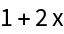In:=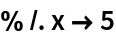Out=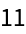If the input expression is in held form, x will not evaluate to any value it might have:

 In:=Out=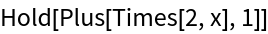The replacement is done before the expression is evaluated, so functions like Set (=) and SetDelayed (:=) work as expected:

 In:=Out=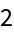The value of x changed:

 In:=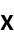Out=### Scope (3)

Convert multiple strings to symbols:

 In:=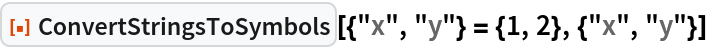Out=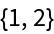In:=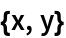Out=Put x in a different context:

 In:=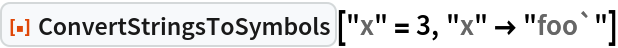Out=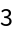In:=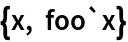Out=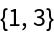Create symbols in different contexts. The context specification Automatic is equivalent to not specifying a context:

 In:=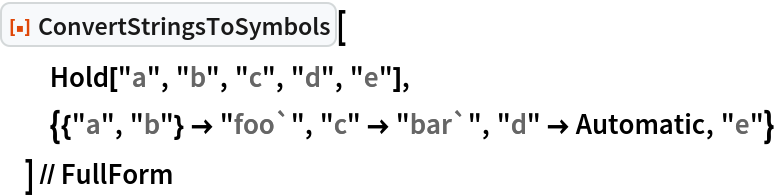Out=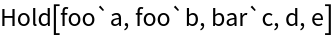### Applications (3)

Construct a range of symbols programmatically and populate them with values:

 In:=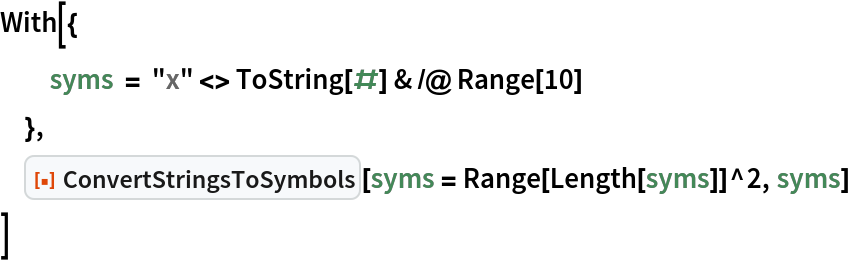Out=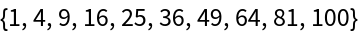Check the values:

 In:=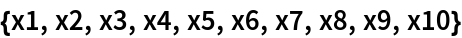Out=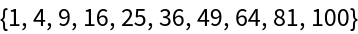This works even when the symbols already have values:

 In:=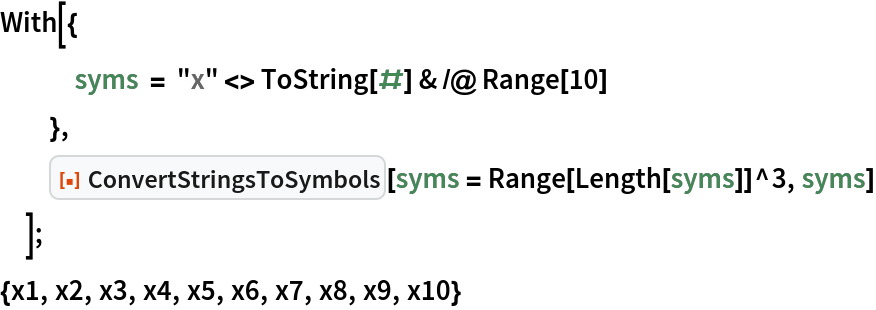Out=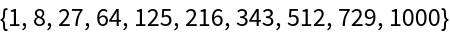Sjoerd Smit

## Version History

• 1.0.0 – 09 May 2022